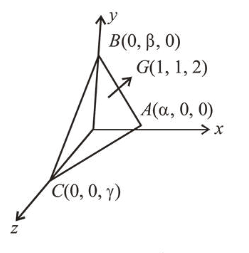# A plane P meets the coordinate axes atQuestion:

A plane $P$ meets the coordinate axes at $A, B$ and $C$ respectively. The centroid of $\triangle \mathrm{ABC}$ is given to be $(1,1,2)$. Then the equation of the line through this centroid and perpendicular to the plane $P$ is:

1. $\frac{x-1}{2}=\frac{y-1}{1}=\frac{z-2}{1}$

2. $\frac{x-1}{1}=\frac{y-1}{1}=\frac{z-2}{2}$

3. $\frac{x-1}{2}=\frac{y-1}{2}=\frac{z-2}{1}$

4. $\frac{x-1}{1}=\frac{y-1}{2}=\frac{z-2}{2}$

Correct Option: , 3

Solution:$\therefore \alpha=3, \beta=3$ and $\gamma=6$ as $\mathrm{G}$ is centroid.

$\therefore$ The equation of plane is

$\frac{x}{\alpha}+\frac{y}{\beta}+\frac{z}{\gamma}=1$

$\Rightarrow \frac{x}{3}+\frac{y}{3}+\frac{z}{6}=1 \Rightarrow 2 x+2 y+z=6$

$\therefore$ The required line is, $\frac{x-1}{2}=\frac{y-1}{2}=\frac{z-2}{1}$